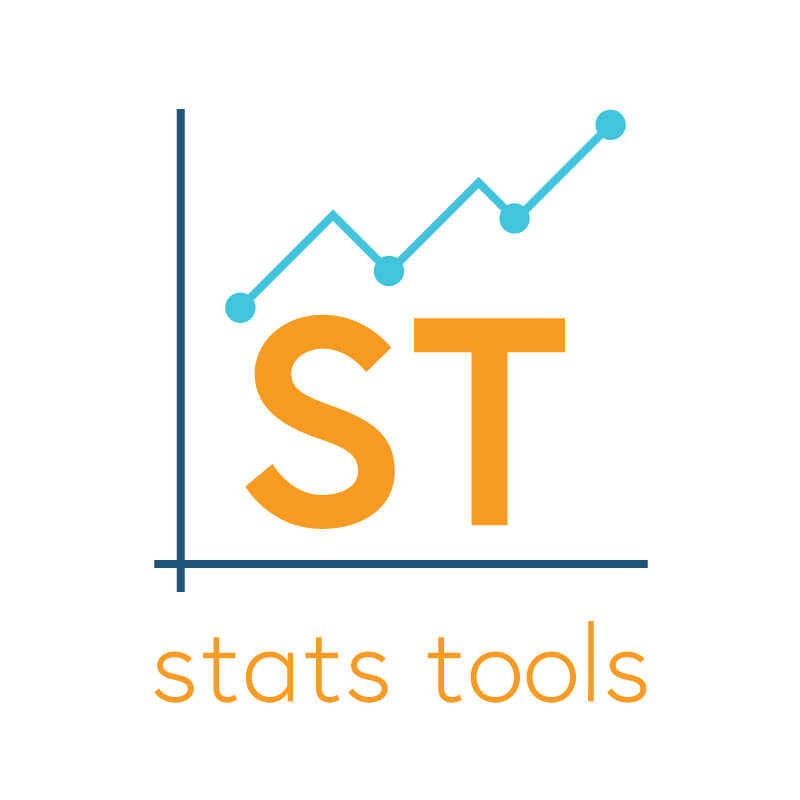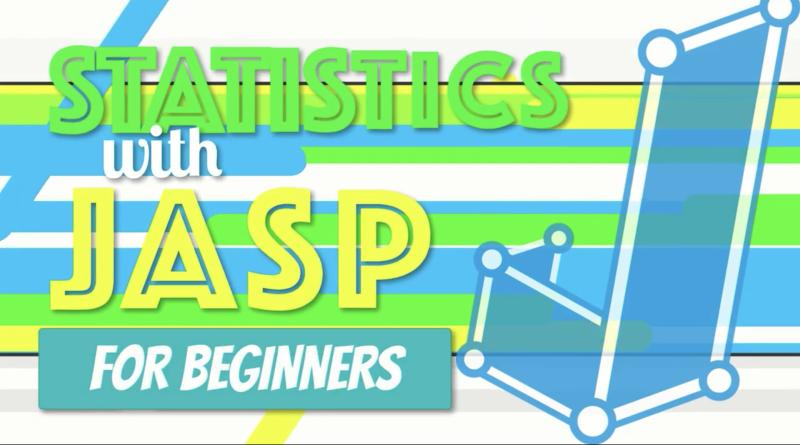# JASP## Resources: Stats of Doom

A comprehensive site created by Dr. Erin Buchanan offering lecture materials and videos for a full range of statistics concepts covered in a basic/introductory course (descriptives, graphs, z-scores, t-tests, ANOVA, correlation, regression, chi-square, etc.). Coverage also includes materials for several statistics packages (e.g., SPSS, JASP, R) Here is a link for a basic course. There …## Video: How to Do Chi-Square in JASP

Video (10:27) from Research by Design in which, “We learn how to calculate a One-Way Chi-Square goodness of fit test in JASP using the setting for Multinomial Test. For the null hypothesis, we assume that the observed values in one group (the Pigs) do not differ from the values in a comparison population (the Bears) …## Video: How to Do Simple Linear Regression in JAS

Video (23:18) from Research by Design in which, “Exploring our data about burnout and job satisfaction, we predict an outcome with a single variable using simple linear regression in JASP. I explain how regression works, then open an SPSS dataset in JASP. We explore the assumptions of homoscedasticity and linearity with a scatterplot and normality …## Video: How to Do a Pearson Correlation in JASP

Video (18:47) from Research by Design in which, “Using a dataset about burnout and job satisfaction, we learn how to conduct a simple correlation, how to interpret it, and how to write it up in APA style. We begin by exploring the theoretical relationship among the variables to understand how correlation works. After we walk …## Video: How to Do an One-Way ANOVA in JASP

Video (14:02) from Research by Design in which, “We are off to see the wizard with an example of a one-way (factorial) ANOVA involving wicked witches and munchkins. We open a .CSV dataset in JASP, check the assumptions of homogeneity of variance and normality, conduct the test, interpret the results, and write up the findings …## Video: How to Do an Independent Samples t Test in JASP

Video (18:29) from Research by Design in which, “Using a dataset about puppy training, we learn how to set up and run an independent samples t test. We import data into JASP, conduct the test, interpret the results and write up the findings. We will learn to check the assumptions of homogeneity of variance using …## Video: How to Do a One Sample and Paired-Samples t Test in JASP

Video (16:57) from Research by Design in which, “The One Sample t-Test is a parametric procedure that tests whether a sample mean is statistically significantly different than a population mean or other known value. We will open a CSV dataset in JASP, check the assumptions of the test, conduct the one sample t-test, interpret the …## Video: Computing Central Tendency and Variability in JASP

Concise video (9:50) from Research by Design in which, “We walk through the steps to identify three measures of central tendency (mean, median, and mode) using JASP. This is an excellent introduction to JASP software and some of the functions that we will use throughout the introductory statistics course.” (7:41) “We will learn how to …## Video: Computing Frequencies and Creating an APA-Style Frequency Table Using JASP, Excel, and Word

Concise video from Research by Design in which, “We create an APA-style frequency table with scores in descending order, simple frequency, relative frequency, cumulative frequency, percentile, and sample size. We use JASP for the statistics, Excel for the formatting, and wrap it all up for presentation in Word. These same techniques work with open-source word …